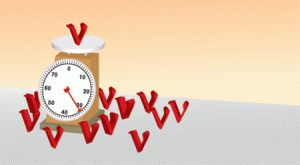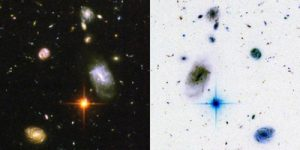## Research goals

The NOvA experiment is designed to answer three fundamental questions in neutrino physics:

### 1. Can we observe the oscillation of muon neutrinos to electron neutrinos?Completely unknown in the macroscopic world, neutrino oscillations are a quantum effect. They are equivalent to a sports car changing into a minivan or a bus, and then, many miles farther down the road, reappearing as a sports car. (Credit: symmetry magazine)

Neutrinos come in three varieties: muon neutrinos, electron neutrinos and tau neutrinos. Scientists know that neutrinos oscillate, or change from one type to another, and have seen, for example, oscillations of muon neutrinos to tau neutrinos. But scientists have not seen muon neutrinos oscillating into electron neutrinos. Unknown factors that govern neutrino oscillations have significant implications for our understanding of the makeup of the universe.

### 2. What is the ordering of the neutrino masses?

Scientists know that the masses of neutrinos are about a million times lighter than the masses of other particles in the Standard Model of physics. However, they do not know the masses of the different neutrino types, nor do they know the neutrino mass hierarchy – that is, which kind of neutrino is the lightest and which is the heaviest. Scientists think that neutrinos get their masses through a different process than the other particles. Therefore they cannot be sure that neutrino masses follow the same pattern as other particles.Physicists think the origins of neutrino masses are closely tied to subatomic processes that took place right after the big bang. Determining which neutrino types are heaviest and lightest—the neutrino mass ordering—is a first step toward revealing these processes. (Credit: symmetry magazine)

Physicists have built various theoretical models to explain the properties of neutrinos. If experimentalists can figure out which mass hierarchy is correct, it will eliminate those models that require a different order.

Knowledge of the mass hierarchy also will help answer the question of whether neutrinos are their own antiparticles. Particles and antiparticles have opposite charges. Because neutrinos have no electric charge, it is possible that neutrinos and antineutrinos are fundamentally the same.

Neutrinos are strange. Scientists describe them either by their flavor (electron, muon or tau) or their masses. To make a neutrino of a particular mass requires a combination of the three flavors.

Neutrinos can have one of three masses. The recipe for the first type of neutrino uses mostly electron flavor. The second type uses roughly equal amounts electron, muon and tau. The third is a nearly 50-50 mixture of muon and tau.

What scientists don’t know is whether this third type of neutrino is the heaviest or the lightest.

Physicists at NOvA hope to determine the mass hierarchy by comparing oscillations of a beam of muon neutrinos to oscillations of a beam of muon antineutrinos.

The normal hierarchy, in which the third type of neutrino is heaviest, tends to favor oscillations of neutrinos over oscillations of antineutrinos. The inverted hierarchy tends to favor the opposite.

### 3. What is the symmetry between matter and antimatter?

If the NOvA collaboration discovers that muon antineutrinos oscillate at a different rate than muon neutrinos, they will know the symmetry between the neutrinos and antineutrinos is broken. This could be a clue to why the universe has more matter than antimatter – the reason we exist.Physicists theorize that the big bang created equal amounts of matter and antimatter.

Physicists theorize that the big bang created equal amounts of matter and antimatter. When corresponding particles of matter and antimatter meet, they annihilate one another. But somehow we’re still here, and antimatter, for the most part, has vanished.

It appears that at some point, matter and antimatter behaved differently from one another.

Physicists once theorized that nothing would change about the laws of physics if every particle were replaced with its antiparticle. This is called charge-parity symmetry. But it turns out that matter and antimatter are not exactly mirror images, and this could explain why they exist in unbalanced quantities. Breaking charge-parity symmetry is called CP violation.

In order to advance the theory that neutrinos tipped the balance between matter and antimatter, neutrino physicists need to observe CP violation in action. Researchers at Fermilab use the NuMI neutrino beam and neutrino detectors to study neutrino and antineutrino oscillation. If antineutrinos do not follow the same pattern as neutrinos when they change from one flavor to another, this is a signal of CP violation. The same mechanism that could cause neutrinos and antineutrinos to oscillate differently could have implications for the mechanism that would have led to an abundance of matter over antimatter in the early universe.